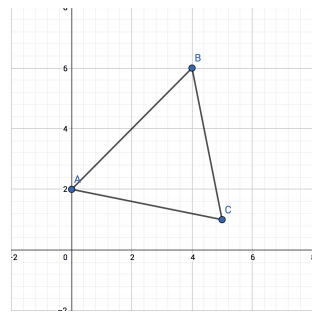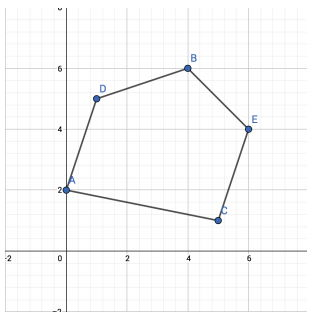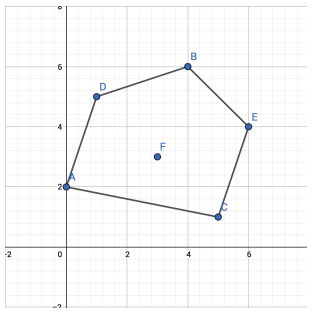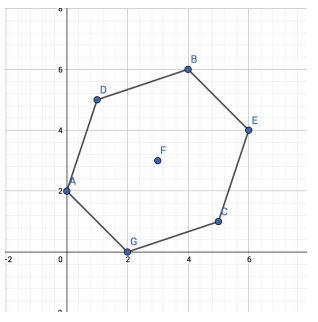시간 제한메모리 제한제출정답맞힌 사람정답 비율
1 초 512 MB8000.000%

## 문제

Let S be a set of points on a 2-dimensional plane. Initially, S is an empty set. Let convexHull(S) be the subset of S, which is the vertices of the convex hull of S without any three collinear points.

There are Q queries, each of the either following type:

1. add(x, y) - Adding a point (x, y) to S. It is guaranteed that no two points share the same xcoordinate or the same y-coordinate.
2. findHeavy(x1, y1, x2, y2) - Point (x1, y1) and point (x2, y2) are guaranteed to be distinct points in convexHull(S). It is also guaranteed that this query is called when convexHull(S) contains at least three points. Suppose a robot is located at (x1, y1) and the robot wants to go to (x2, y2). He can only traverse between the edges of the convex hull of S. Let cw be the number of points in convexHull(S) traversed by the robot if the robot moves in clockwise direction, and ccw be the number of points in convexHull(S) traversed by the robot if the robot moves in counter-clockwise direction. If cw ≥ ccw, output "CW", otherwise output "CCW".

## 입력

The first line contains an integer: Q (1 ≤ Q ≤ 100,000) denoting the number of queries. The next Q following lines, each contains three or five integers.

• If the i-th query is an add(x, y) query, then the i-th line will be: 0 x y (0 ≤ x, y ≤ 1,000,000).
• If the i-th query is a findHeavy(x1, y1, x2, y2) query, then the i-th line will be: 1 x1 y1 x2 y2 (0 ≤ x1, y1, x2, y2 ≤ 1,000,000).

## 출력

For each findHeavy(x1, y1, x2, y2) query, output the answer of the query on its own line.

## 예제 입력 1

14
0 0 2
0 4 6
0 5 1
1 0 2 4 6
1 4 6 0 2
0 1 5
0 6 4
1 0 2 4 6
1 0 2 5 1
0 3 3
1 0 2 6 4
0 2 0
1 0 2 6 4
1 6 4 0 2


## 예제 출력 1

CCW
CW
CCW
CW
CW
CW
CW


The following points are added to S after the first three queries, as well as the convex hull.For the fourth query, cw is 1 while ccw is 2, thus, we print “CCW".

For the fifth query, cw is 2 while ccw is 1, thus, we print "CW".

The following points are added to S after the first seven queries, as well as the convex hull.For the eight query, cw is 2 while ccw is 3, thus, we print "CCW".

For the ninth query, cw is 4 while ccw is 1, thus, we print “CW".

The following points are added to S after the first ten queries, as well as the convex hull.For the eleventh query, cw is 4 while ccw is 1, thus, we print “CW".

The following points are added to S after the first twelve queries, as well as the convex hull.For the thirteenth query, cw is 3 while ccw is 3, thus, we print "CW".

For the fourteenth query, cw is 3 while ccw is 3, thus, we print "CW".